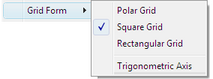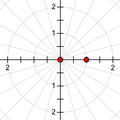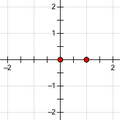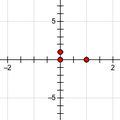This Graph menu command changes the grid appearance and scaling of the marked coordinate system. Sketchpad’s coordinate systems can have several possible forms:Polar Grid: A polar coordinate system has a set of grid lines that are circles (at a constant distance from the origin, or r value) and a set of grid lines that pass through the origin (at a constant angle from the origin, or θ value). Any coordinate system can be made polar. If the horizontal and vertical axes of a polar coordinate system have different scaling, the constant-distance grid lines appear as ellipses rather than circles.Polar Square Grid: A square coordinate system has the same scaling on the horizontal and vertical axes and has grid lines that are horizontal (at a constant y value) and vertical (at a constant x value). Any coordinate system, except one defined in terms of two different distances, can be made square.Square Rectangular Grid: A rectangular coordinate system has independent scaling for the horizontal and vertical axes and has grid lines that are horizontal (constant y value) and vertical (constant x value). Any coordinate system, except one defined in terms of a unit circle, can be made rectangular.Rectangular Trigonometric Axis: The horizontal axis is numbered with fractions and multiples of π. You can use a trigonometric axis on any kind of coordinate system. When you choose trigonometric axes for a polar grid, both axes become trigonometric. You can turn trigonometric numbering on or off for any axis by selecting it and choosingSquare with Trigonometric Axis